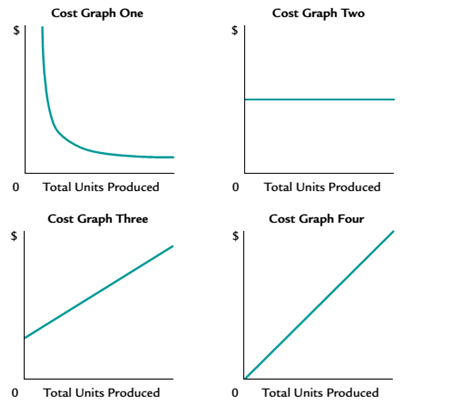Chapter 11, Problem 11.2E

Chapter
Section
Textbook Problem

Identify cost graphs The following cost graphs illustrate various types of cost behavior:For each of the following costs, identify the cost graph that best illustrates its cost behavior as the number of units produced increases. a. Direct material cost per unit. b. Fees for using a patent of \$500,000 plus \$0.25 for each unit produced. c. Salary of quality control supervisor. d. Straight-line depreciation per unit on factory equipment. e. Total direct materials cost.

To determine

Concept Introduction:

Cost Graph: Cost graph is a diagram which shows the different possible combination of the cost on various level of the production. It shows the different combination of the cost on different level of production.

To Identify:

Prescribe the cost graph for the different costs.

Explanation

a. Direct material cost per unit= Cost graph two because direct material cost remains same on every level of production.

b. Fees for using a patent of \$ 500,000 plus .25 for each unit produced= Cost graph three because it is fixed till a particular level and increases thereafter.

c...

Still sussing out bartleby?

Check out a sample textbook solution.

See a sample solution

The Solution to Your Study Problems

Bartleby provides explanations to thousands of textbook problems written by our experts, many with advanced degrees!

Get Started

What is an ERP system?

Accounting Information Systems

Discuss the dirrerence between budgets and standard costs.

Managerial Accounting: The Cornerstone of Business Decision-Making

RATIO CALCULATIONS Assume the following relationships for the Brauer Corp.: Sales/Total assets 1.5x Return on a...

Fundamentals of Financial Management, Concise Edition (with Thomson ONE - Business School Edition, 1 term (6 months) Printed Access Card) (MindTap Course List)

Give an example of each.

Foundations of Business (MindTap Course List)

What are the three strategic themes of the financial perspective?

Cornerstones of Cost Management (Cornerstones Series)